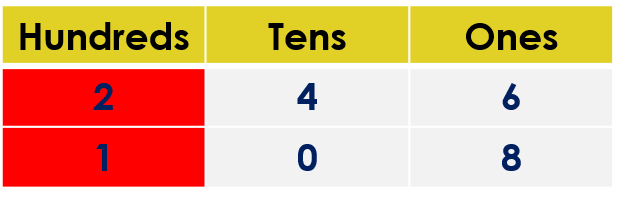1
visibility
Fill in the blank space with the correct sign. Use either <, > or =.
246  \$latex \framebox[0.5in]{}\$  108

The correct answer is ">".

It is easy!

• Compare the hundreds digits.
• If the hundreds digits are the same, then compare the tens digits.
• If tens digits are the same, then compare the ones digits.

Solution:

Observe that both numbers have three digits.

246 ____ 108

Comparing the hundreds digits:We observe that both digits are NOT the same.

• The hundreds digit in the number 246 is 2.
• The hundreds digit in the number 108 is 1.

But, 2 is greater than 1.

So, 246 is greater than 108.

That is, 246 > 108.